# Capacitor Converter DC Circuit Breaker with Current Limiting Function

Capacitor Converter DC Circuit Breaker with Current Limiting Function
School of Automation and Electrical Engineering, Lanzhou Jiaotong University, Lanzhou 730070, China

Corresponding Author Email:
2419123252@qq.com
Page:
139-147
|
DOI:
https://doi.org/10.18280/ejee.240303
19 April 2022
|
Accepted:
7 June 2022
|
Published:
30 June 2022
| Citation

OPEN ACCESS

Abstract:

DC circuit breaker is the core equipment for the safe and stable operation of DC transmission system. In order to design a DC circuit breaker with low cost and current limiting function, a capacitor converter DC circuit breaker with current limiting function is proposed in this paper. The topology uses capacitance to replace IGBT to realize the switching of circuit state, which greatly reduces the number of IGBT, moreover, through the use of current limiting branch, the peak value and rate of current are restrained, the requirements of energy absorption capacity of lightning arrester are reduced, the fault cut-off time is shortened, and the cost and technical difficulty are greatly reduced. In this paper, the working principle of the extracted topology is studied and analyzed in stages, and then the parameters are designed. Finally, the system simulation model is built by using PSCAD software to verify the simulation model. Compared with the typical circuit breaker design scheme, the rationality and superiority of the proposed scheme are proved.

Keywords:

capacitor converter, current limiting function, DC transmission, DC circuit breaker

1. Introduction

At present, the flexible DC power grid technology of modular multilevel converter (MMC) has gradually developed into one of the important choices of new energy power generation . Overhead line transmission is the main transmission mode of DC Power Grid Group . With the increasing application of new energy power generation year by year, the scale of HVDC transmission system continues to expand because HVDC can more effectively improve the utilization efficiency of renewable new energy . However, due to the increasingly complex structure of DC transmission system, it has brought great potential safety hazards to the operation of the system and converter station . As the core equipment to protect the safe and stable operation of DC system, the impedance of DC power grid is very low. In case of fault, the current will rise rapidly within a few milliseconds, which seriously threatens the safety of power grid. Therefore, the fault must be removed quickly and effectively .

High voltage DC circuit breakers are divided into mechanical DC circuit breakers, all solid state DC circuit breakers and Hybrid DC circuit breakers . Hybrid DCCB combines the first two technologies, which can perfectly meet the requirements of fast DC breaking speed and reduce DC on state loss . In 2012, abb of Switzerland researched and manufactured Hybrid DC circuit breaker for the first time . In 2014, the global energy Internet Research Institute developed a hybrid DCCB based on fast mechanical switch and full bridge module cascade, and the circuit breaker was applied in Zhoushan flexible DC transmission project in 2016 . In 2019, in the experiment of Zhangbei flexible DC power grid, the DC circuit breaker with voltage level up to 535 kV passed the acceptance test . Since then, various types of hybrid HVDC circuit breaker topologies have been proposed one after another.

Due to the increasing voltage of DC power transmission, the application of fault current limiter is proposed in order to reduce the current borne by the circuit breaker during fault . The current limiter can not only limit the rise rate of fault current after fault, but also reduce the peak value of fault current, so as to reduce the current capacity borne by the circuit breaker. The resistance current limiter proposed in Ref.  applies superconducting technology. Due to the high cost of superconducting technology and high requirements in practical application, it is difficult to be applied to engineering. The topologies proposed by Zhang et al.  are all added with pre charging capacitors. After the fault current is cut off, the voltage polarity of the capacitor changes, but it is difficult to restore its original polarity. Therefore, pre charging is required when reclosing. The working principle of the multi branch current limiting circuit breaker proposed in the literature [14, 15] is that when the line fails, the current inductance is converted from parallel to series to realize the current limiting capacity, and the current limiting effect is good. However, because it is divided into multiple branches, the number of IGBTs and the cost of circuit breakers soar. Moreover, a large number of IGBTs in series have the problem of dynamic voltage equalization, which makes the scheme more difficult ; The H-bridge structure adopted in Ref.  not only enables the circuit breaker to realize bidirectional shutdown, but also halves the number of IGBT used, but also suppresses the rise rate and peak value of fault current. However, a large number of IGBT devices need to be connected in series and parallel, and the cost is still high.

In order to better reduce the cost of DC circuit breaker and strengthen the current limiting capacity of circuit breaker, a capacitor converter DC circuit breaker (CCL-DCCB) topology with current limiting function is proposed in this paper. The topology uses the converter capacitor to replace the IGBT of the transfer branch in the traditional Hybrid DC circuit breaker, and the topology uses the bypass branch to reduce the energy absorbed by the arrester. The current limiting branch is designed in the form of the combination of resistance, inductance and capacitance to effectively suppress the fault current. In addition, the current limiting branch can realize internal self charging without configuring charging equipment.

2. Topology and Working Principle

2.1 Topology

1) Current conducting branch: it is composed of ultra fast mechanical switch (UFMS) and load transfer switch (LCS). When the circuit operates normally, the only path of current.

2) Current limiting branch: it is composed of four groups of thyristors, a group of freewheeling diode D, capacitor C1, current limiting inductance L and current limiting resistance RL. The suppression of the circuit current is realized by the charge and discharge of capacitor C1 and the limitation of inductance L on the current.

3) Breaking current branch: it is composed of energy discharge branch composed of energy discharge resistance R2, thyristor group T5, converter capacitor C2, metal oxide arrester MOV, thyristor T5 and resistance R2 in series in parallel. This part is used to carry and break the fault current, and use the lightning arrester to dissipate the energy on the non fault side.

4) Drainage current branch: it is composed of unloading resistor Rby and unloading thyristor Tby in series. When the arrester mov starts to act, the fault side inductance Ldc is removed by the bypass, and the energy unloading resistance Rby in the bypass branch absorbs and dissipates the energy on the fault side, reducing the energy absorbed by the arrester MOV (Figure 1).

## 1.png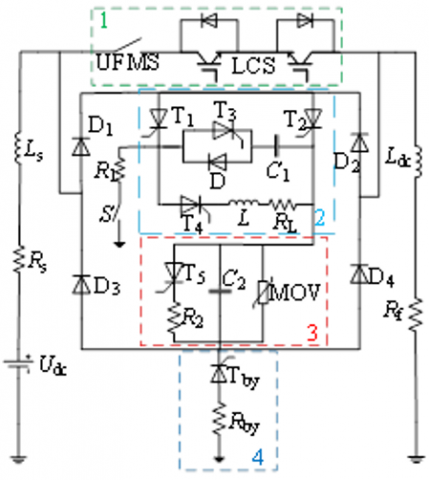Figure 1. CCL-DCCB topological structure

2.2 Working principle

According to the Ref. , due to the small error, MMC can be simplified as the series equivalent of power supply Udc, resistance Rs and inductance Ls. The fault current transfer path of CCL-DCCB is shown in Figure 2. The flat wave reactance is Ldc, and the equivalent resistance is expressed in Rf.

1) Pre-charge process (t0<t<t1)

From t0 to t1, the DC system operates stably and normally. During this period, the initial charging of capacitor C1 is completed through the conduction of T2 and S. The charging principle diagram of capacitor C1 is shown in Figure 2(a). The function of large resistance R1 is to limit the charging current value. When the capacitor C1 is charged, the current flowing through T2 decreases to zero and T2 is successfully turned off. At this point, the pre-charge of capacitor C1 is completed.

## 2a.png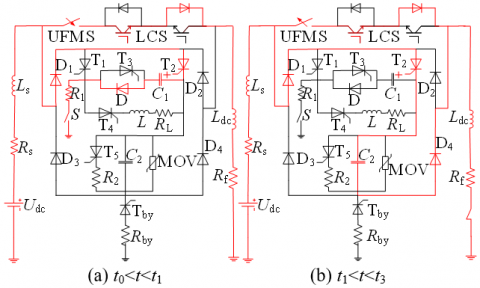## 2b.png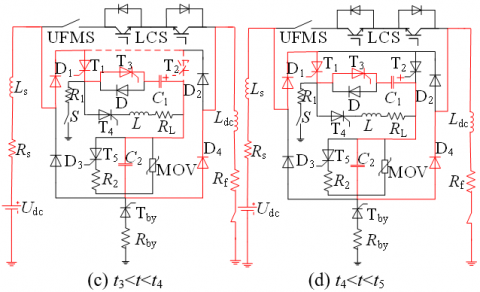## 2c.png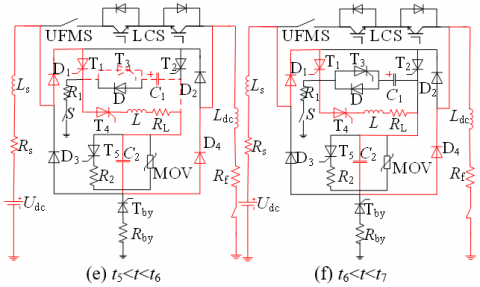## 2d.png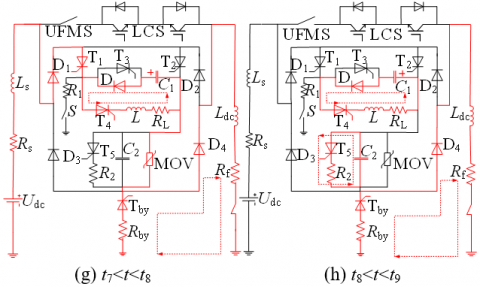Figure 2. Fault current transfer path of CCL-DCCB

2) Current limiting preparation process (t1<t<t3)

When a fault occurs at t1, the current of the through-current branch increases rapidly. Since no fault is detected, the through-current branch is still on, and the other branches are still in the off bypass state. At time t2, a short-circuit fault is detected, the LCS in the current branch is quickly shut down, and a shut-off signal is given to the UFMS, and the UFMS starts to open distance. Turn on T2 in the current passing branch and the converter capacitor C2 in the current breaking branch, the fault current begins to transfer from the current passing branch to the circuit breaker, and the converter capacitor C2 begins to charge, ensuring that the current branch does not need to bear high voltage. At time t3, UFMS reaches the rated opening distance (generally 2 ms). The current path in this period is shown in Figure 2(b).

(t1<t<t2): during t1 to t2 time period, the DC line fails, but due to the delay, only the smoothing reactance Ldc suppresses the fault current. According to KVL, the equation can be obtained:

${{U}_{\text{dc}}}={{L}_{\text{0}}}\frac{\text{d}{{i}_{\text{dc}}}}{\text{d}t}+{{R}_{\text{0}}}{{i}_{\text{dc}}}$                 (1)

The initial value of idc in the equation is the current value before the fault occurs, that is idc(t0)=In, The solution is as follows:

$i_{\mathrm{dc}}=\frac{U_{\mathrm{dc}}}{R_{0}}\left(I_{\mathrm{n}}-e^{-\left(t-t_{0}\right) / \tau}\right)$                         (2)

where: $\tau=L_{0} / R_{0} ; R_{0}=R_{\mathrm{s}}+R_{\mathrm{f}} ; L_{0}=L_{\mathrm{s}}+L_{\mathrm{dc}}$.

(t2<t<t3): At time t2, the conduction of converter capacitor C2 can be obtained from the equation according to KVL:

$\left\{\begin{array}{l}U_{\mathrm{dc}}=L_{0} \frac{\mathrm{d} i_{\mathrm{dc}}}{\mathrm{d} t}+R_{0} i_{\mathrm{dc}}+u_{\mathrm{C}_{2}} \\ i_{\mathrm{C}_{2}}=i_{\mathrm{dc}}=C_{2} \frac{d u_{\mathrm{C}_{2}}}{d t}\end{array}\right.$          (3)

It can be seen from equation (2) that the current value of the line is idc(t2)=iC2(t2)=I2, which can be obtained by substituting into Eq. (3):

\begin{aligned} i_{\mathrm{C}_{2}}=i_{\mathrm{dc}}=& e^{-\alpha\left(t-t_{2}\right)}\left(U_{\mathrm{dc}} C_{1} \omega \sin \left(\omega\left(t-t_{2}\right)\right)\right.\left.-I_{2} \sin \left(\omega\left(t-t_{2}\right)-\beta\right)\right) \end{aligned}              (4)

where:

$\alpha=R_{s} / 2\left(L_{s}+L_{\mathrm{dc}}\right) ; \beta=\arctan (\omega / \alpha)$;

$\omega=1 / \sqrt{C_{2} L_{0}} ; R_{0}=R_{s}+R_{f} ; L_{0}=L_{s}+L_{\mathrm{dc}}$.

3) Current limiting process (t3<t<t8)

(t3<t<t4): At time t3, after the UFMS is fully open, the signal is sent to T1, and T1 immediately turns on (T2 has been successfully turned on at time t3. By analyzing the circuit composed of C1, T3, T1 and T2, it can be seen that T1 is successfully turned on after giving T1 turn on signal because T1 bears positive pressure before turning on), the capacitor C1 and puts it into operation. Since the anode voltage direction of capacitor C1 is opposite to the short-circuit current direction, the capacitor discharges first, the voltage released by capacitor C1 makes T3 turn on, and T2 turns to bear the back voltage. Therefore, the initial anode current of T2 decreases rapidly, and the current flowing to T2 is gradually transferred to T1. Until t4, the current flowing through T2 decreases to zero, and T2 is successfully turned off by T1. The current path in this period is shown in Figure 2(c).

(t4<t<t5): At t4, T2 is turned off, but the discharge of capacitor C1 is not over. At t5, the discharge of converter capacitor C1 is over. The current path in this period is shown in Figure 2(d).

The dynamic process of C1 discharge is described as follows:

$\left\{\begin{array}{l}U_{\mathrm{dc}}=L_{0} \frac{\mathrm{d} i_{\mathrm{C}_{1}}}{\mathrm{~d} t}+R_{0} i_{\mathrm{C}_{1}}-u_{\mathrm{C}_{1}}+u_{\mathrm{C}_{2}} \\ i_{\mathrm{C}_{1}}=i_{\mathrm{dc}}=i_{\mathrm{C}_{2}}=-C_{1} \frac{\mathrm{d} U_{\mathrm{C}_{1}}}{\mathrm{~d} t}\end{array}\right.$             (5)

Let the initial charging value of capacitor C1 be the voltage value U0 during pre-charge. Since T2 turns off rapidly, it can be ignored, that is, it is considered that all the current is transferred to T3 branch at t3 time. The initial value of the current flowing through the capacitor C1 is the current value obtained by taking the time t3 into Eq. (4). Assuming that the current value i(t3)=idc(t3)=I3 at this time, substituting into the Eq. (5), it can be solved that the capacitor voltage and capacitor branch current in this stage are:

$U_{\mathrm{C}_{1}}=\left(U_{\mathrm{dc}}+U_{\mathrm{C}_{1}}\right) \cos \left(\gamma\left(t-t_{3}\right)\right)-\frac{I_{3}}{C_{1} \gamma} \sin \left(\gamma\left(t-t_{3}\right)\right)-U_{\mathrm{dc}}$                    (6)

\begin{aligned} i_{\mathrm{C}_{1}}=&\left(U_{\mathrm{dc}}+U_{\mathrm{c}_{1}}\right) C_{1} \gamma \sin \left(\gamma\left(t-t_{3}\right)\right) \\ &+I_{3} \cos \left(\gamma\left(t-t_{3}\right)\right) \end{aligned}                     (7)

where, $\gamma=\sqrt{\left(\frac{\left.C_{1}+C_{2}\right)}{C_{1} C_{2} L_{0}}\right.} ; L_{0}=L_{s}+L_{\mathrm{dc}}$.

(t5<t<t6): At t5, the discharge of capacitor C1 ends. At this time, the initial voltage value of capacitor C1 is 0, that is uC1(t5)=0.

$\left\{\begin{array}{l}U_{\mathrm{dc}}=L_{0} \frac{\mathrm{d} i_{\mathrm{C} 1}}{\mathrm{~d} t}+R_{\mathrm{L}} i_{\mathrm{L}}+u_{\mathrm{C}_{1}}+u_{\mathrm{C}_{2}} \\ u_{\mathrm{C} 1}=-L \frac{\mathrm{d} i_{\mathrm{L}}}{\mathrm{d} t}-R_{\mathrm{L}} i_{\mathrm{L}} \\ i_{\mathrm{L}}=-C_{1} \frac{\mathrm{d} u_{\mathrm{C} 1}}{\mathrm{~d} t} \\ i_{\mathrm{C}_{2}}=i_{\mathrm{dc}}=i_{\mathrm{C} 1}+i_{\mathrm{L}}\end{array}\right.$                            (8)

Simplification:

$\frac{C_{1} C_{2}}{C_{1}+C_{2}} L_{0} \frac{\mathrm{d}^{2} u_{\mathrm{C}}}{\mathrm{d} t^{2}}+\left(\frac{L_{0}+L}{L}\right) u_{\mathrm{C}}+U_{\mathrm{dc}}=0$         (9)

According to Eq. (7), the initial value of current at t5 is iC1(t5)=idc(t5)=I5. Substitute the initial value UC1(t5)=0 into the Eq. (8).

\left\{\begin{aligned} i_{\mathrm{C}_{1}}=& A C_{1} \lambda \sin \left[\lambda\left(t-t_{5}\right)\right]+I_{5} \cos \left[\lambda\left(t-t_{5}\right)\right] \\ u_{\mathrm{C}_{1}}=& \frac{-I_{5}}{\lambda C_{1}} \sin \left[\lambda\left(t-t_{5}\right)\right]+A\left[\cos \lambda\left(t-t_{5}\right)-1\right] \\ i_{\mathrm{L}}=&-\frac{A}{\lambda}\left[\sin \left(\lambda\left(t-t_{5}\right)\right)-\lambda\left(t-t_{5}\right)\right] \\ &+B\left[1-\cos \left(\lambda\left(t-t_{5}\right)\right)\right] \end{aligned}\right.                      (10)

where: $A=\sqrt{L U_{\mathrm{dc}} /\left(L+L_{0}\right)} ; C=\frac{C_{1} C_{2}}{\left(C_{1}+C_{2}\right)} ; B=\frac{I_{5}}{L C} \lambda^{2} ; \lambda=$ $\sqrt{\left(L+L_{0}\right) / L_{0} L C} ; L_{0}=L_{s}+L_{\text {dc }}$.

With the reverse charging of capacitor C1, the longer the charging time, the smaller the current IC1 flowing through capacitor C1 and the greater the voltage uC1. At time t6, uc1 rises to the system voltage and capacitor C1 is disconnected. The current path in this period is shown in Figure 2(e).

(t6<t<t7): After t6, the resistance sensing branch is fully put into operation. According to Eq. (10), the initial value of current at t6 is idc(t6) =iL(t6)=I6. From KVL and KCL, the instantaneous current IL in the resistance sensing branch is:

$i_{\mathrm{L}}=I_{6} e^{-\left(t-t_{6}\right) / \tau_{0}}+\frac{U_{\mathrm{dc}}}{R_{\Sigma}}\left(1-e^{-\left(t-t_{6}\right) / \tau_{0}}\right)$                   (11)

where: $\tau_{0}=\left(L_{0}+L\right) / R_{L} ; R_{\Sigma}=R_{L}+R_{s}+R_{f}$.

When the current limiting impedance L branch is turned on, the current rise rate decreases. The current path in this period is shown in Figure 2(f).

4) Cut off process (t7<t<t8)

At t7, when the arrester mov reaches the working voltage, the arrester MOV is turned on and operated, the converter capacitor C2 is turned off, and the drainage branch is turned on and grounded. After the arrester MOV is put into operation, it starts to absorb the energy on the non fault side and limit the overvoltage. The reactance on the fault side begins to discharge to the grounding resistance Rby through the thyristor Tby of the drainage branch. The operation of the drainage branch reduces the energy consumption demand of the arrester. The capacitor C1 forms a charge discharge circuit through diode D, current limiting inductance and current limiting resistance. The capacitor C1 is discharged through the circuit first and then charged, so that the capacitor current returns to the state after pre-charge, so that it is not necessary to recharge the capacitor C1 for each subsequent disconnection fault current. At t8, the current flowing through the arrester is zero, the energy absorption of the arrester is completed, and the fault line is cut off successfully. The current path in this period is shown in Figure 2(g).

The recording t7~t8 period is the energy absorption time of the arrester Δt. According to Eq. (11), the initial value of current at t7 is idc(t7)=iL(t7)=I7, and the action voltage is UMOV, which is obtained from KVL:

$i_{\mathrm{dc}}=I_{7} e^{-\Delta t / \tau_{1}}+\frac{U_{\mathrm{dc}}-U_{\mathrm{MOV}}}{R_{\mathrm{s}}+R_{\mathrm{L}}}\left(1-e^{-\Delta t / \tau_{1}}\right)$                  (12)

The solution is as follows:

$\Delta t=-\frac{L+L_{\mathrm{s}}}{R_{\mathrm{L}}+R_{\mathrm{s}}} \ln \frac{\Delta U}{\left(R_{\mathrm{L}}+R_{\mathrm{s}}\right) I_{7}-\Delta U}$                  (13)

where: $\tau_{1}=\left(L+L_{s}\right) /\left(R_{L}+R_{s}\right) ; \Delta U=U_{\mathrm{dc}}-U_{\mathrm{MOV}}$.

5) Energy release process (t8<t<t9)

At t8, the energy absorption of the arrester ends and the current on the non fault side drops to zero. The energy on the fault side is exhausted through the grounding resistance Rby. At the same time, the capacitor C1 also continues to charge and discharge through the diode D and the resistance sensing branch to form a discharge circuit. Turn on the signal to the thyristor T5, and the converter capacitor C2 discharges through the energy discharge resistance R2. At time t9, all circuits are completed. The current path in this period is shown in Figure 2 (h).

When the capacitor C1 is charged and discharged through the diode D with the current limiting inductance and current limiting resistance, there is no oscillation process due to the unidirectional conductivity of the diode. From Eq. (10), uC1(t6)=U6, from Eq. (11), idc(t7)=iL(t7)=I7, the KVL equation is:

$L C_{1} \frac{d^{2} U_{\mathrm{C}_{1}}}{d t^{2}}+R_{\mathrm{L}} C_{1} \frac{d U_{\mathrm{C}_{1}}}{d t}+U_{\mathrm{C}_{1}}=0$                (14)

The charging current of the capacitor is:

$i_{\mathrm{C}_{1}}=\theta \sqrt{\frac{C_{1}}{L}} e^{-\tau_{2}\left(t-t_{7}\right)} \sin (\chi t+\delta)$               (15)

where: $\tau_{2}=\frac{R_{L}}{2 L} \quad ; \quad \chi=\sqrt{\frac{1}{L C_{1}-\frac{R_{L}^{2}}{4 L^{2}}}}$; $\theta=\sqrt{U_{7}^{2}+\left(\tau_{2} U_{7} / \chi-I_{7} / \chi C_{1}\right)^{2}}$$\sigma=\arctan \left[U_{7} /\left(\tau_{2} U_{7} \chi-I_{7} / \chi C_{1}\right)\right]$.

3. Device Parameter Design

The equivalent rated voltage of DC power grid in this paper is 500 kV. The device selection and analysis of circuit breaker are based on the voltage of 500 kV. In this section, the current limiting inductance L, current limiting resistance RL, discharge resistance R2 and capacitors C1 and C2 of the circuit breaker are analyzed to ensure that CCL-DCCB can realize optimal shutdown.

3.1 Parameter design of current limiting inductance L

The use of current limiting reactance in the circuit suppresses the rising rate of current. The larger the reactance, the more obvious the suppression effect, but it will prolong the time when the current limiting inductor L is fully put into operation and prolong the fault breaking time. The current limiting inductor L is put into operation from t5, fully operational at t6. At this time, iC=0 is brought into Eq. (10) to obtain the time required for the resistance sensing branch to be fully put into the fault circuit Δt0 is:

$\Delta t_{0}=t_{6}-t_{5}=\frac{\pi-\eta}{\rho}$               (16)

where: $\eta=\arctan \left[\frac{I_{4}\left(L_{0}+L\right)\left(C_{1}+C_{2}\right)}{U_{\mathrm{dc}} L C_{1} C_{2} \rho}\right] ; \rho=\frac{1}{\sqrt{L_{0} C_{2}}}$.

According to the analytical formula (16), The value of Δt0 is affected by inductance L, capacitance C1 and C2. When it is determined that the values of capacitors C1 and C2 remain unchanged, it can be seen that the value of Δt0 will increase with the increase of inductance L; Moreover, when the fault current flowing through it reaches a steady state, the larger the reactance L, the more energy stored by the reactance L itself. Figure 3(a) is obtained according to Eq. (16), Δt0 follows the variation law of current limiting inductance L.

## 3.png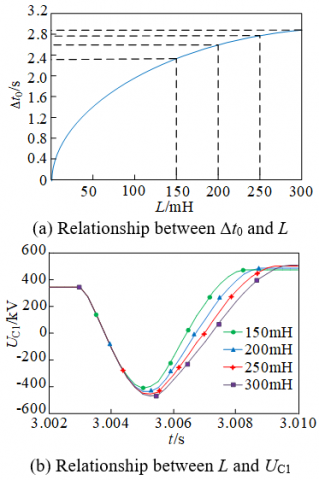Figure 3. Influence of inductance L value

Since the size of smoothing reactor Ldc currently used in the project is 150 mH , combined with the fact that the rated voltage of DC power grid is 500 kV, the actual value of given current limiting inductance is between 150-300 mH. As shown in Figure 3(b), the larger the value of inductance L, the longer the time required for capacitor C1 to reverse charge to the highest voltage. This shows that the larger the inductance value, the longer the time it takes for the branch where the current limiting inductance is fully put into operation, and the greater the voltage value of capacitor C1; And the high-voltage reactance is expensive. Therefore, for 500 kV DC system, the resistance inductance branch adopts 200 MH current limiting inductance.

3.2 Parameter design of current limiting resistance RL

The current limiting resistor RL limits the rising amplitude of fault current after the resistance sensing branch is turned on; In the circuit composed of current limiting inductor L and capacitor C1, the energy of L is dispersed into RL and C1. By changing the resistance value of current limiting resistor RL, the charging voltage and circuit discharge time constant of C1 can be adjusted. As can be seen from Figure 4(a), the resistance value of RL is inversely proportional to the voltage of capacitor C1; The greater the resistance, the smaller the capacitor voltage. It can be seen from Figure 4(b) that the size of resistance RL has no obvious effect on the size of fault current. And for high-power resistors, it is necessary to consider the limitations of their own volume and heat dissipation performance, so the resistance value cannot be increased indefinitely. Therefore, the current limiting resistor with resistance value of 10 Ω is adopted in this scheme.

## 4.png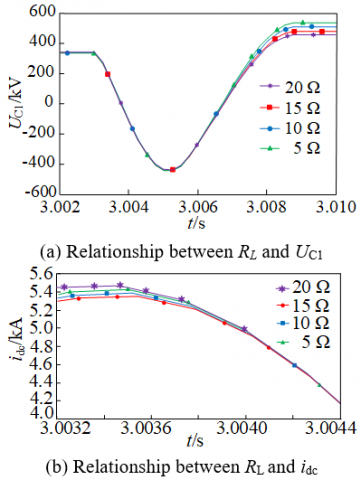Figure 4. Influence of resistance RL value

3.3 Parameter design of capacitor C1 and C2

3.3.1 Parameter design of capacitor C1

When designing the value of capacitor C1, the withstand voltage capacity of the capacitor during charging should be considered first. The CCL-DCCB topology proposed in this paper controls the on-off of the branch where capacitor C1 is located through thyristor T1(T2), and the charge and discharge of capacitor C1 also controls the off of thyristor T2(T1). Therefore, the voltage that capacitor C1 can withstand is greater than the peak value of capacitor reverse voltage UC1m1. The relationship between the peak value of reverse voltage UC1m1 and the value of capacitance C1 is shown in Figure 5(a). Secondly, it is necessary to consider the second charging process of capacitor C1 through the resistance inductance branch When all the energy in the current limiting inductor is transferred to the capacitor, the capacitor voltage reaches the peak UC1m2. The relationship between the capacitance voltage peak UC1m2 and the capacitance C1 value is shown in Figure 5(a). It can be seen from the figure that the value of capacitance should be 10 μF-20 μF.

This paper takes 500kV DC system as the research object. By comparing the changes of line current when different capacitance values change in Figure 5(b), The smaller the C1 value of the capacitor, the faster the charging of the capacitor, the lower the peak current, the shorter the time required for the branch where the resistance and reactance are fully put into operation, and the shorter the time required for the shutdown of the fault line. Considering comprehensively, the value of capacitance C1 is 10 μF.

## 5.pngFigure 5. Influence of capacitance C1 value

3.3.2 Parameter design of capacitor C2

The converter capacitor C2 is the key equipment to complete the switching in the circuit breaker design. When the voltage at both ends of C2 reaches the MOV starting voltage, the current is transferred to MOV and the converter capacitor C2 is disconnected. Therefore, the voltage of the whole circuit breaker is the starting voltage of MOV. Set the MOV starting voltage as Utig, and the size of Utig has a great impact on the converter capacitor C2. The larger the Utig, the higher the voltage peak of the converter capacitor C2, the higher the requirements for the withstand voltage level of the capacitor, and the cost increases accordingly; The higher the Utig, the longer the charging time of capacitor C2 and the longer the off time of circuit breaker. In practical engineering application, the starting voltage of the arrester is 1.6 times of the rated voltage , that is Utig = 1.6Udc. Before the arrester reaches the starting voltage, the instantaneous voltage of the converter capacitor C2 is shown in Eq. (17).

$U_{\mathrm{C}_{2}}(t)=\frac{1}{C_{2}} \int_{t_{2}}^{t} i_{\mathrm{dc}}(t) d t \quad t_{2}<t<t_{7}$            (17)

It can be seen from Eq. (17) that if Utig is within 5 ms , the upper limit of capacitance C2 brought in by system parameters UC2-max=30 μF. The traditional hybrid DCCB requires Utig to be less than 5 ms because the traditional hybrid DCCB uses IGBT to turn off the current, and the continuous growth of the current will cause permanent damage to power electronic devices. Although CCL-DCCB mentioned in this text replaces C2 with converter capacitor, since the whole circuit breaker has other power electronic devices, in order to ensure the normal use of all devices, the selection of converter capacitor should be within 30 μF. As can be seen from Figure 6, the larger the value of capacitor C2, the longer the starting time of arrester, the larger the value of fault current, and the longer the time that the fault line is turned off. However, the smaller the value of capacitor C2, the greater the voltage stress of power electronic valve group in the circuit. Considering comprehensively, the converter capacitor C2 is taken as 30 μF.

## 6.png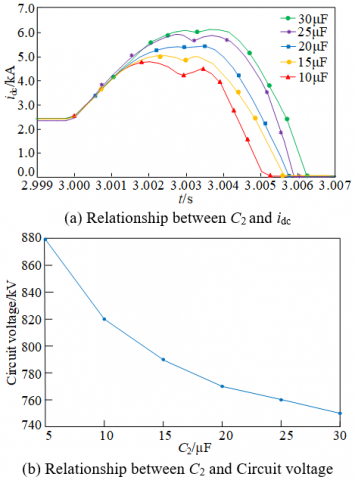Figure 6. Influence of capacitance C2 value

3.4 Parameter design of energy discharge resistance R2

## 7.png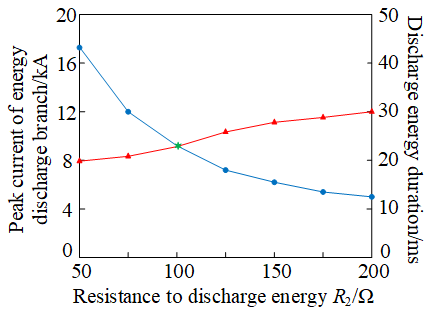Figure 7. Influence of energy discharge resistance R2 value

After the fault is successfully isolated, the thyristor T5 is turned on and the converter capacitor C2 is discharged through the energy discharge resistance R2. When the current flowing through the energy discharge resistance circuit decreases to zero, the thyristor T5 is disconnected and the CCL-DCCB returns to the initial state. The greater the value of R2, the less likely the overcurrent phenomenon of the circuit is. However, the greater the value of R2, the longer the discharge time of the converter capacitor C2. Due to the limitation of its own volume and heat dissipation performance, the resistance value cannot be improved indefinitely. Therefore, the peak current should also be considered when designing resistance R2. The variation of peak current and discharge time of energy discharge branch with energy discharge resistance R2 is shown in Figure 7. As can be seen from Figure 7, the peak current of the energy discharge branch is inversely proportional to the size of the energy discharge resistance R2, but the energy discharge time is directly proportional to the size of the energy discharge resistance R2. Therefore, when selecting the energy discharge resistance R2, its resistance value cannot be too small or too large. As can be seen from Figure 7, the energy discharge resistance at the intersection of the two curves is R2=100 Ω, so the value of energy discharge resistance in this paper is 100 Ω.

4. Simulation Analysis

4.1 Simulation system parameters

Figure 8 shows the simulation model of four terminal flexible DC power grid built through PSCAD, and its parameters are shown in Table 1. When selecting various devices, first consider the actual models produced by ABB. The rated voltage and current of IGBT is 4.5 kV/3 kA, the rated voltage and current of thyristor is 8.5 kV/4.5 kA, and the rated voltage and current of diode is 4.5 kV/3.8 kA. In the CCL-DCCB topology, the current limiting capacitor C1 is designed as 10 μF. The current limiting resistance RL is designed to be 10 Ω, the current limiting inductance L is designed to be 200 mH, and the converter capacitor C2 is designed to be 20 μF. The energy discharge resistance R2 is designed to be 150 Ω.

## 8.png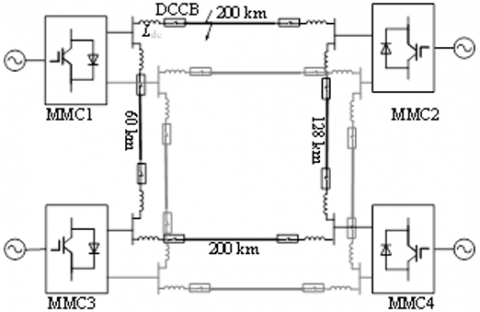Figure 8. Simulation model of DC power grid

Table 1. Simulation parameters of DC power grid

 System parameter MMC1 MMC2 MMC3 MMC4 Converter capacity/MW 1500 500 3000 1500 Sub module capacitance/mF 7 15 15 7 Bridge arm inductance/mH 100 50 50 100 Reactance level/mH 150 150 150 150

4.2 Fault clearing simulation

Figure 9 shows the current transfer diagram of CCL-DCCB breaking fault in case of fault.

When t=3.0 s (time t1), the system has a short-circuit fault. After a delay of 1 ms, the fault is detected when t= 3.001 s (time t2) and the current transfer begins. The LCS is turned off immediately, the thyristor T2 and the converter capacitor C2 are turned on at the same time, and the UFMS starts to open. After 2 ms, i.e. when t =3.0030 s (time t3), UFMS reaches the rated opening distance and completely turns off, and gives T3 conduction signal at the same time. At this time, T1 is turned on under the forward voltage and the capacitor C1 begins to discharge. When t=3.0031 s (time t4), T2 is turned off due to backpressure. When t=3.0033 s (time t5), the discharge of capacitor C1 ends, reverse charging begins, T4 is turned on, and the branch where current limiting reactance L is located is put into use. At time t=3.0045 s (time t6), the branch where the current limiting reactance L is located is fully put into operation, the capacitor branch is turned off, and the current rising speed is reduced. When t=3.0049 s (time t7), the voltage at both ends of the arrester reaches the opening voltage, the arrester starts to consume energy, and the converter capacitor C2 is bypassed; At the same time, the thyristor Tby of the bypass branch is on, and the bypass branch consumes the energy on the fault side; The circuit composed of resistance sensing branch and capacitor C1 starts to charge the capacitor. When t=3.0057 s (time t8), the fault side current is 0 and the circuit fault is cleared; Bypass branch resistance Rby still consumes energy at fault side; The circuit composed of resistance sensing branch and capacitor C1 is still charging the capacitor; At the same time, the thyristor T5 is turned on, and the converter capacitor C2 begins to discharge through the energy discharge resistance R2 until 3.009 s (time t9).

## 9.png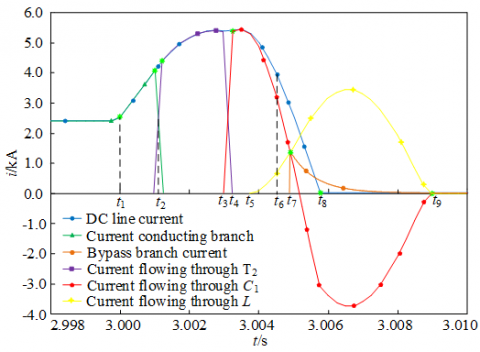Figure 9. Simulation waveform

5. Performance Comparison

In order to objectively analyze the breaking performance of the circuit breaker proposed in this paper, this paper is simulated and compared with other schemes. Scheme 1 is the traditional hybrid DCCB proposed by ABB in document , and scheme 2 is the current limiting hybrid HVDC circuit breaker proposed in document , scheme 3 is the capacitor converter DC circuit breaker proposed in document , and scheme 4 is the capacitor converter DC circuit breaker with current limiting function proposed in this scheme. The obtained simulation comparison diagram is shown in Figure 10.

Scheme one has no ability to suppress the fault current. Before the arrester does not act, the fault current increases rapidly to the peak value. Moreover, since the output current cannot be effectively controlled, the fault elimination time is also long, and it takes 10 ms; At the same time, the energy absorption of the arrester is increased by 7.5 ms, and the turn-off time of the arrester is significantly reduced; The biggest difference between scheme 3 and schemes 1 and 2 is that it replaces IGBT power electronic devices with capacitors in the current breaking branch, which saves the cost, but the current peak value and turn-off time are improved compared with scheme 1, and the turn-off time is reduced by 1 ms to 9.0 ms compared with scheme 1; In scheme 4, on the basis of replacing IGBT with capacitor, a current limiting device is added to the circuit breaker to reduce the amplitude of breaking current, and a bypass current branch is added. Since the energy stored in the current limiting inductor is transferred to the current limiting capacitor and resistance, the energy absorbed by the arrester is reduced, the breaking speed of the circuit breaker is enhanced, and the closing time is 5.8 ms.

## 10.png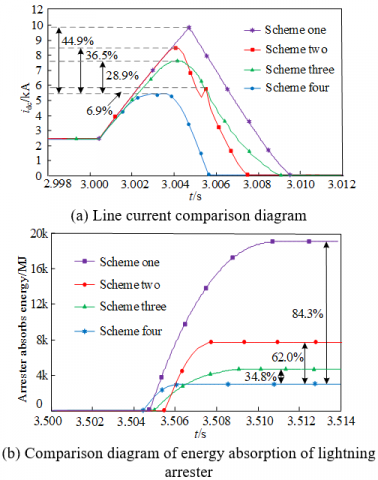Figure 10. Schemes comparison

Compared with scheme one, the peak current of scheme 4 decreases by 44.9%; Compared with scheme two phase scheme, the peak current decreases by 44.9%; Compared with the three-phase scheme, the peak current drop is 28.9%. Due to the same reference voltage of the arrester, the energy absorbed by the arrester in the scheme proposed in this paper is 84.3% lower than that in scheme one; Scheme two reduced by 62.0%; It is 34.8% lower than scheme three. However, compared with the other three schemes, the discharge time of the arrester is very short, and it can quickly recover to the rated voltage of the system after the energy absorption process. To sum up, the scheme proposed in this paper has positive significance for the development of DC circuit breaker. The comparative analysis of breaking performance parameters of the four schemes is shown in Table 2.

By comparing the number of devices used in each scheme and the energy absorption of lightning arrester, the device specifications selected in scheme 1, 2 and 3 are the same as those in this paper.

In the scheme described in this paper, all IGBT are applied to the through flow current branch. The pressure of the IGBT valve group is 22.5 kV and the through flow is 4.05 kA. 1 UFMS, 10 IGBT and 10 diodes are required. T1 and T4 thyristor valve groups in the current limiting branch bear 800 kV, and T2 and T3 thyristor valve groups bear 500 kV; the pressure bearing capacity of thyristor valve group in bypass branch is 500 kV; the pressure of thyristor valve group in the cut-off branch is 800 kV. The current demand of all thyristors is 5.4 kA, and the number of thyristors required is 924. In the scheme, the pressure bearing of diodes distributed on the bridge circuit is 800 kV, but the pressure bearing of diodes in the circuit composed of resistance sensing branch and capacitor C1 is 500 kV, so the number of diodes required is 1252. Therefore, the scheme requires a total of 1 UFMS, 10 IGBT, 924 thyristors and 1262 diodes. The number of devices required for each scheme is shown in Table 2.

In terms of technical difficulty, the failure rate of a single IGBT is 0.0119 times/cycle, and there is a problem of dynamic voltage sharing in large-scale IGBT Series. The circuit breaker described in this paper replaces IGBT with capacitor, and the fault rate of capacitor is 0.000916 times/cycle, which is lower than that of IGBT. Almost all the circuit breakers use thyristors to complete line breaking, and the thyristor series parallel technology is mature, so the technical difficulty of large-scale use is lower than that of large-scale use of IGBT. In addition, in terms of the cost of the device itself, the price of IGBT is 5 times that of thyristor and 10 times that of diode . Therefore, this scheme is high in cost performance, reliability and stability.

Table 2. Simulation parameters

 Project Scheme one Scheme two Scheme three Scheme four Number of IGBT/piece 1424 884 10 10 Number of thyristors/piece 0 627 2577 924 Number of diodes/piece 10 0 10 1262 MOV energy consumption /MJ 19.1 7.9 4.6 3.0
6. Conclusions

After analyzing the structure, principle, parameter design and simulation verification of CCL-DCCB proposed in this paper, the following conclusions are obtained.

1) CCL-DCCB replaces the traditional hybrid IGBT with converter capacitor. Compared with the traditional Hybrid DC circuit breaker, the number of IGBT is sharply reduced, and the energy consumption of lightning arrester is reduced by 84.3%, greatly reducing the cost.

2) CCL-DCCB topology uses the thyristor as the switching device, adopts the bridge structure to realize the two-way shutdown, realizes the pre charging of the circuit breaker through the DC power grid, and the addition of the current limiting device reduces the peak value of the fault current, which has good economic performance and lays a good foundation for the large-scale use of the circuit breaker.

3) The CCL-DCCB topology proposed in this paper makes full use of the power supply and charges the converter capacitor through the power supply equipment of the circuit. Moreover, the capacitor is charged through the circuit of capacitor and resistance sensing branch, so that the capacitor can be reused indefinitely after only one charge, which solves the technical problem that it is necessary to configure charging device for pre-energy storage capacitor separately, so as to effectively reduce the volume and cost of the whole circuit breaker.

References

 Zhang, F.X., Guo, X.S., Chang, Z.T., Zhang, K. (2020). Short-time current test method of key equipment for Zhangbei DC grid. High Voltage Engineering, 46(2): 682-689. https://doi.org/10.13336/j.1003-6520.hve.20190321005

 Wang, Y.H., Fu, Y.T., Zeng, Qi., Song, Y.Y. (2019). Review on key techniques for fault protection of flexible DC grids. High Voltage Engineering, 45(8): 2362-2374. https://doi.org/10.13336/j.1003-6520.hve.20190731002

 Arman, H., Jurgen, H., Bjorn, J. (2014). Technical assessment of load converter switch in hybrid HVDC breaker. IEEE Transactions on Power Electronics, 30(10): 5393-5400. https://doi.org/10.1109/TPEL.2014.2372815

 Xu, Z., Xiao H.Q., Xu Y.Z. (2018). Study on basic principle and its realization methods for DC circuit breakers. High Voltage Engineering, 44(2): 347-357. https://doi.org/10.13336/j.1003-6520.hve.20180131002

 Huang, Q., Zou G.B., Gao, L., Song, C.J. (2018). Review on DC transmission line protection technologies of HB-MMC based DC grids. Power System Technology, 42(9): 2830-2840. https://doi.org/10.13335/j.1000-3673.pst.2017.2593

 Wang, Z.H., Tian, Q., Cheng, L., Li, G.Q. (2019). Transient breaking characteristics and its parameter influence analysis of hybrid DC circuit breaker. Power System Protection and Control, 47(18): 1-10. https://doi.org/10.19783/j.cnki.pspc.181229

 He, J.J. (2019). Research on Key Technologies of High Voltage DC Circuit Breaker. High Voltage Engineering, 45(8): 2353-2361. https://doi.org/10.13336/j.1003-6520.hve.20190731001

 Jovcic, D., Zaja, M., Hedayati, M.H. (2020). Bidirectional hybrid HVDC CB with a single HV valve. IEEE Transactions on Power Delivery, 55(1): 269-277. https://doi.org/10.1109/TPWRD.2019.2912832

 Qiu, P., huang, X.M., Wang, Y. (2018). Application of high voltage DC circuit breaker in Zhoushan VSC-HVDC transmission project. High Voltage Engineering, 44(2): 403-408. https://doi.org/10.13336/j.1003-6520.hve.20180131009

 Liu, C.Y., Wang, Q.L., Chai, W.Q. (2020). Prototype development and experimental study of ±535kV hybrid DC circuit breaker applied in Zhangbei four-terminal flexible straight project. High Voltage Engineering, 46(10): 3638-3646. https://doi.org/10.13336/j.1003-6520.hve.20191080

 Gupta, R., Barker, C.D., Adamczyk, A., Whitehouse, R.S., Dyke, K.J. (2016). Protection coordination in multi-terminal HVDC networks for DC fault clearance. 13th International Conference on Development in Power System Protection 2016 (DPSP), pp. 1-6, https://doi.org/10.1049/cp.2016.0050

 Willam, R., Pascal, T., Bertrand, R. (2017). Technical and economic analysis of the R-type SFCL for HVDC grids protection. IEEE Transactions on Applied Superconductivity, 27(7): 1-9. https://doi.org/10.1109/TASC.2017.2739642

 Zhang, C.L., Wu D.Y., Chen, K. (2019). DC fault current limiter based on pre charge commutation capacitor. Proceedings of the CSEE, 39(S1): 54-62. https://doi.org/10.13334/j.0258-8013.pcsee.190807

 Han, N.Z., Jia, X.F., Zhao, X.B. (2019). A novel hybrid DC fault current limiter topology. Proceedings of the CSEE, 39(6): 1647-1658. https://doi.org/10.13334/j.0258-8013.pcsee.180469

 Nie, Z.P., Yu, Z.Q., Gan, Z.Z. (2021). Topology modeling and design of a novel magnetic coupling fault current limiter for VSC DC grids. IEEE Transactions on Power Electronics, 36(4): 4029-4041. https://doi.org/10.1109/TPEL.2020.3025461

 Zhang, X.J., Huang, M., Liu, J.H. (2021). Review of voltage balancing methods for series-connected IGBTs. Journal of Power Supply, 19(1): 192-204. https://doi.org/10.13234/j.issn.2095-2805.2021.1.192

 Tang, S., Jia, G.L., Zhan, C.H. (2020). Topology of DC circuit breaker with pre-current-limiting capability for DC grid. Automation of Power Systems, 44(11): 152-168.

 Wang, J.J., Wang, Z.X. (2019). A novel hybrid high-voltage DC circuit breaker topology with current limiting capability. Electric Power Automation Equipment, 39(10): 143-149. https://doi.org/10.16081/j.epae.201909009

 Li, S., Xu, J., Lu, Y., Zhao, C., Zhang, J., Jiang, C., Qiu, S. (2019). An auxiliary DC circuit breaker utilizing an augmented MMC. IEEE Transactions on Power Delivery, 34(2): 561-571. https://doi.org/10.1109/TPWRD.2019.2892445

 Callavik, M., Blomberg, A., Hafner, J., Jacobson, B. (2012). The hybrid HVDC breaker: an innovation breakthrough enabling reliable HVDC grids. ABB Grid Systems.

 Wang, Z.H., Zhang, L.B., Chen, L. (2021). A hybrid HVDC circuit breaker with current limiting function. Power Grid Technology, 45(5): 2042-2049. https://doi.org/10.13335/j.1000-3673.pst.2020.0654

 Jiang, C.B., Zhao, C.Y. (2021). Capacitor converter DC circuit breaker with reclosing judgment ability. In 2021 High Voltage Technology, 47(9): 3254-3263. https://doi.org/10.13336/j.1003-6520.hve.20210234

 Zhang, S., Zou, G.B., Wei, X.Y. (2021). A review of research on multi-port DC circuit breaker. Proceedings of the CSEE, 41(13): 4502-4516. https://doi.org/10.13334/j.0258-8013.pcsee.201514